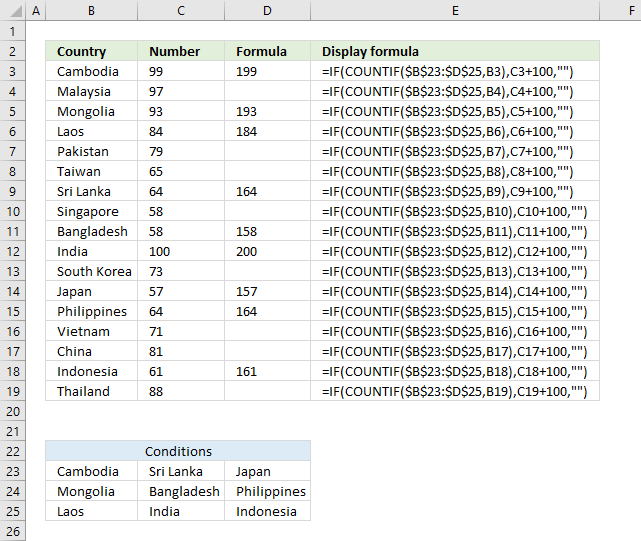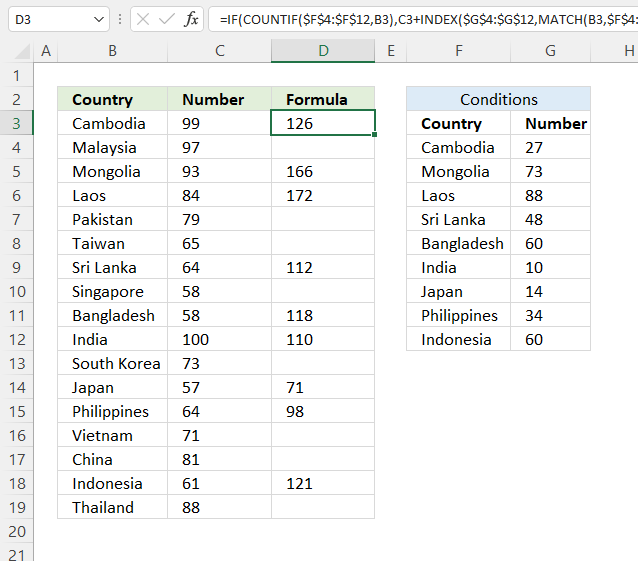Author: Oscar Cronquist Article last updated on September 17, 2021The image above demonstrates a formula that matches a value to multiple conditions, if the condition is met the formula takes the value in a corresponding cell on the same row and adds a given number.

The COUNTIF function allows you to construct a small IF formula that carries out plenty of logical expressions.

Combining the IF and COUNTIF functions also let you have more than 254 logical expressions and the effort to type the formula is minimal.

## 1. Use IF + COUNTIF to evaluate multiple conditions=IF(COUNTIF(\$B\$23:\$D\$25,B3),C3+100,"")

The example shown in the above picture checks if the country in cell B3 is equal to one of the countries in cell range B23:D25.

In other words, the COUNTIF function counts how many times a specific value is found in a cell range.

If the value exists at least once in the cell range the IF function adds 100 to the value in C3. If FALSE the formula returns a blank.

### 1.1 Explaining formula in cell D3

#### Step 1 - COUNTIF function syntax

The COUNTIF function calculates the number of cells that is equal to a condition.

COUNTIF(rangecriteria)

#### Step 2 - Populate COUNTIF function arguments

COUNTIF(rangecriteria)

becomes

COUNTIF(\$B\$23:\$D\$25,B3)

range - A reference to all conditions: \$B\$23:\$D\$25

criteria - The value to match.

#### Step 3 - Evaluate COUNTIF function

COUNTIF(\$B\$23:\$D\$25,B3)

becomes

COUNTIF({"Cambodia", "Sri Lanka", "Japan"; "Mongolia", "Bangladesh", "Philippines"; "Laos", "India", "Indonesia"}, "Cambodia")

and returns 1. The criteria value is found once in the array (bolded).

#### Step 4 - IF function syntax

The IF function returns one value if the logical test is TRUE and another value if the logical test is FALSE.

IF(logical_test, [value_if_true], [value_if_false])

#### Step 5 - Populate IF function arguments

IF(logical_test, [value_if_true], [value_if_false])

becomes

IF(1, C3+100, "")

logical_test - True or False, the numerical equivalents are TRUE - 1 and False - 0 (zero). 1, in this case, is equal to TRUE.

[value_if_true] - C3+100, add 100 to value in cell C3.

[value_if_false] - "".

#### Step 6 - Evaluate IF function

IF(COUNTIF(\$B\$23:\$D\$25, B3), C3+100, "")

becomes

IF(1, C3+100, "")

becomes

C3 + 100

becomes

99 + 100

and returns 199 in cell D3.

## 2. Use IF + COUNTIF to evaluate multiple conditions and calculate different outcomesThe image above demonstrates a formula in cell D3 that checks if the value in cell B3 matches any of the conditions specified in cell range F4:F12. If so, add the corresponding number in cell range G4:G12 to the number in cell C3.

Formula in cell D3:

=IF(COUNTIF(\$F\$4:\$F\$12, B3), C3+INDEX(\$G\$4:\$G\$12, MATCH(B3, \$F\$4:\$F\$12,0)), "")

### 2.1 Explaining formula

#### Step 1 - Check if the value matches any of the conditions

The COUNTIF function calculates the number of cells that is equal to a condition.

COUNTIF(rangecriteria)

COUNTIF(\$F\$4:\$F\$12, B3)

becomes

COUNTIF({"Cambodia"; "Mongolia"; "Laos"; "Sri Lanka"; "Bangladesh"; "India"; "Japan"; "Philippines"; "Indonesia"}, "Cambodia")

and returns 1. This means that there is one value that matches.

#### Step 2 - IF function

The IF function returns one value if the logical test is TRUE and another value if the logical test is FALSE.

IF(logical_test, [value_if_true], [value_if_false])

IF(COUNTIF(\$F\$4:\$F\$12, B3), [value_if_true], [value_if_false])

becomes

IF(1, [value_if_true], [value_if_false])

[value_if_true] - C3+INDEX(\$G\$4:\$G\$12, MATCH(B3, \$F\$4:\$F\$12,0))

[value_if_false] - ""

#### Step 3 - Calculate the relative position of a lookup value

The MATCH function returns the relative position of an item in an array or cell reference that matches a specified value in a specific order.

MATCH(lookup_value, lookup_array, [match_type])

MATCH(B3, \$F\$4:\$F\$12,0)

becomes

MATCH("Cambodia", {"Cambodia"; "Mongolia"; "Laos"; "Sri Lanka"; "Bangladesh"; "India"; "Japan"; "Philippines"; "Indonesia"}, 0)

and returns 1. The lookup value is found at the first position in the array.

#### Step 3 - Get value

The INDEX function returns a value from a cell range, you specify which value based on a row and column number.

INDEX(array, [row_num], [column_num])

INDEX(\$G\$4:\$G\$12, MATCH(B3, \$F\$4:\$F\$12,0))

becomes

INDEX(\$G\$4:\$G\$12, 1)

and returns 27.

#### Step 4 - Add values

The plus sign lets you add numbers in an Excel formula.

C3+INDEX(\$G\$4:\$G\$12, MATCH(B3, \$F\$4:\$F\$12,0))

becomes

99 + 27 equals 126.